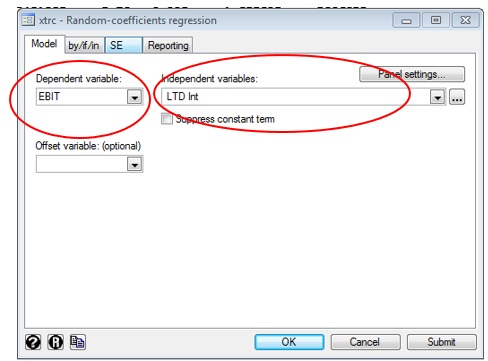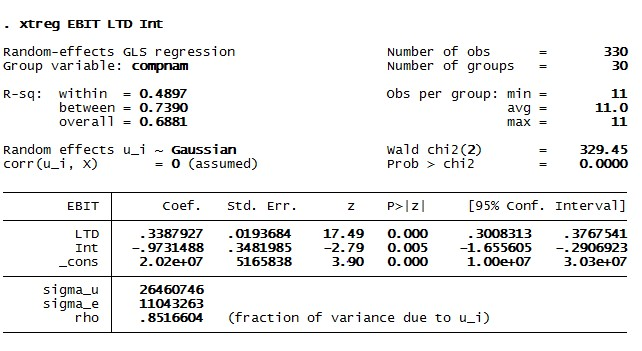# How to perform Panel data regression for random effect model in STATA?

The previous article (Pooled panel data regression in STATA) showed how to conduct pooled regression analysis with dummies of 30 American companies. The results revealed that the joint hypothesis of dummies reject the null hypothesis that these companies do not have any alternative or joint effects. Therefore pooled regression is not a favourable technique for the panel data sets. It is also due to the fact that inclusion of too many dummies can lead to consequent loss of degrees of freedom. Therefore pooled regression is not the right technique to analyze panel data series. Therefore the present article intends to introduce to the concept of random effect model in STATA.

## Ways to conduct panel data regression

There are two ways to conduct panel data regression; random effects model and fixed effect model. In a random effect model, the intercepts in the regression equation represent the mean values of cross-sectional intercepts. On the other hand, the error terms represent random deviations of individual intercepts from the mean value. Therefore internalise the effects of different cross sections (in this case, 30 firms) as random effects in the regression equation. Since no joint or alternative effect appears in regression results, generate the effect estimates.

## Conducting random effect model in STATA

Use the below command to start with random effect panel data analysis:

`xtreg EBIT LTD Int, re`

In the above syntax, “xtreg” represents the command Earnings Before Interest and Taxes (EBIT), Long Term Debt (LTD) and Interest payments (INT). These are the variables in the order of dependent and independent variables. “re” represents the random effects for the above panel data regression. The below results will appear.Figure 1: Result of the random effect model in STATA for panel data analysis

In the above results, both the independent variables have significant effects on the dependent variable EBIT.  Therefore,

1. The coefficients of independent variable LDT and INT are significant with the p-value less than 0.05.
2. The R square value also indicates the goodness of fit equals 68% which is significantly large.
3. The rho value is 0.8516 which indicates the individual effects of cross-sections are 0.8%.

Since the data set is not normal, there are several implications in the random effect model. Although non-normal data in case of panel regression does not have any direct impact when observations are above 83, it may have some indirect impacts. Due to lack of normality, the error variances may be unequal and the problem of heteroscedasticity may be present. To check this, use the below command.

`xttest0`

The below result will appear.Figure 2: Heteroscedasticity in panel data regression for random effect model in STATA

The above figure represents the outcome of Breusch and Pegan Lagrangian Multiplier test which helps to identify the presence of heteroscedasticity. The null hypothesis for above test is homoscedasticity and alternative hypothesis suggest heteroscedasticity. Since the p values are 0.000 reject the null hypothesis. Thus the above random effect model contains the problem of heteroscedasticity.

## Correcting heteroscedasticity in the random effect model in STATA

In order to rectify the heteroscedasticity use another version of the random effect model known as ‘random effect with GLS’. Follow the below steps.

1. Click on ‘Statistics’ in the main window.
2. Go to ‘Longitudinal/ panel data’.
3. Click on ‘Random coefficients regression by GLS’.

Or use the below STATA command.

`xtrc EBIT LTD Int`Figure 3: STATA pathway for random GLS model

The below window will appear. Here select the dependent and independent variables.Figure 4: Conducting random effect GLS model in STATA

The results of the above GLS random effect model will appear.Figure 5: Results of the GLS random effect model in STATA

The above results show that the model takes care of the problem of heteroscedasticity Also the variable interest does not appear to be significantly different from the previous model. The overall random effects model looks fine and indicates that the relationship between concerned variables as significant.

However, the results do not indicate if the randomness of this random effect model is correct and if it provides the effect set of solution. In order to determine that, it is necessary to perform the fixed effects model in the next article.

### Saptarshi Basu Roy Choudhury

Senior Research Analyst at Project Guru
Saptarshi has done his M. Phil in International Trade and Development and Masters in Economics from Jawaharlal Nehru University, New Delhi. His academic interests include issues related to economics of climate change, regulation and contemporary trade theories. He has a keen interest in current affairs and likes to read and travel in his spare time.

#### Latest posts by Saptarshi Basu Roy Choudhury (see all)

Discussions

1.Olusola

2.has